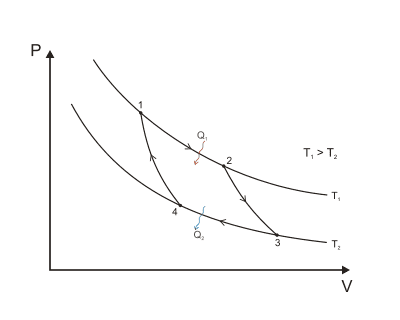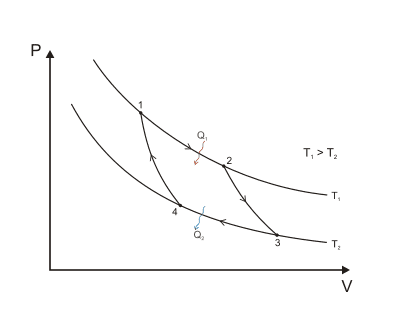# Carnot Cycle Question

• max0005

## Homework Statement

Dear All,

I have a question regarding the Carnot Cycle:(The image is taken from Wikipedia, I hope that is not a problem... In case it is I will change it.)

Points one to two are an isothermal reaction. The gas, kept at constant temperature, is expanding, the work done by the gas is positive, the volume is increasing and, consequently, the pressure is decreasing.

Points two to three are an adiabatic reaction. There is basically no work done either on or from the gas. However, as there still is a small increase in volume but no heat exchange with the outside there will be a significant decrease in pressure. (Question: Is such a small change in volume in an adiabatic expansions able to create such a change in pressure?)

Points three to four are an isothermal compression. Work is done against the gas (Work of the gas is negative) but pressure remains constant. For that to occur, I believe that heat should be flowing out of the system? How is that happening? Theoretically by compressing a gas both its pressure and its internal energy should be rising, but that doesn't seem to be the case. How is heat being "exported" such that the pressure can remain low enough for the work needed to compress the gas to be sufficiently low?

Points four to one are a second adiabatic compression. A small compression is applied but as heat is no longer being removed pressure is increasing. Again, the work done on the gas is negligible.

My question is the one stated in the third paragraph: How is heat being "exported" such that the pressure can remain low enough for the work needed to compress the gas to be sufficiently low?

## The Attempt at a Solution

You seem to have a lot of misconceptions.

## Homework Statement

Dear All,

I have a question regarding the Carnot Cycle:(The image is taken from Wikipedia, I hope that is not a problem... In case it is I will change it.)

Points one to two are an isothermal reaction. The gas, kept at constant temperature, is expanding, the work done by the gas is positive, the volume is increasing and, consequently, the pressure is decreasing.

Points two to three are an adiabatic reaction. There is basically no work done either on or from the gas. However, as there still is a small increase in volume but no heat exchange with the outside there will be a significant decrease in pressure. (Question: Is such a small change in volume in an adiabatic expansions able to create such a change in pressure?)
If there is a change in volume, there is work being done on or by the gas. Since Q=0 for an adiabatic process, the change in internal energy is equal to the work done on the gas.
Points three to four are an isothermal compression. Work is done against the gas (Work of the gas is negative) but pressure remains constant. For that to occur, I believe that heat should be flowing out of the system? How is that happening? Theoretically by compressing a gas both its pressure and its internal energy should be rising, but that doesn't seem to be the case. How is heat being "exported" such that the pressure can remain low enough for the work needed to compress the gas to be sufficiently low?
How do you get that the pressure is constant? From the diagram, you can clearly see it's not constant. Also, I'm not sure why you think heat can't flow out of the system. The gas is being compressed, so the work done on the gas is adding energy to the system. Since the process is isothermic, the internal energy of the gas isn't changing, and all of the energy added by the work must simultaneously be leaving the system via heat loss.
Points four to one are a second adiabatic compression. A small compression is applied but as heat is no longer being removed pressure is increasing. Again, the work done on the gas is negligible.
How are you getting that the work is negligible?
My question is the one stated in the third paragraph: How is heat being "exported" such that the pressure can remain low enough for the work needed to compress the gas to be sufficiently low?